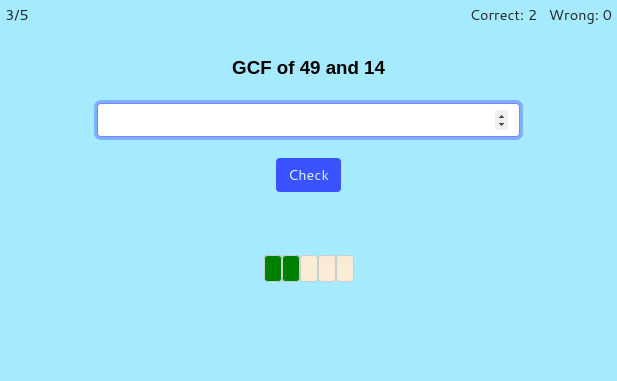MM Practice
×
Multiplication
Division
Place Value
Fractions & Decimals
Measurement
Statistics & Probability
Pre-Algebra
Money
Kindergarten
Geometry

# Greatest common factor (GCF) and least common multiple (LCM)Online practice for grades 5-8

On this page, you can practice finding the greatest common factor (GCF) and the least common multiple (LCM) of two, three, or four numbers.

You can choose timed or untimed practice, the number of practice problems, and the quantity of numbers. There's also an option for "easy mode", which uses smaller numbers than the regular mode.

numbers

Screenshot:OR
Practice for a set time: min

Allow my comment to be posted on this site